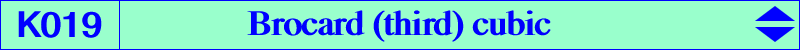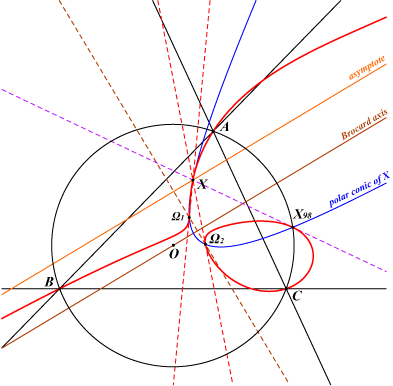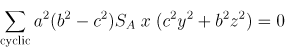X(98), X(511), X(10221), X(13414), X(13415), X(16068), X(16069), X(16070), X(16071), X(32481), X(32482), X(39641), X(39642) Brocard points Ω1 and Ω2 X(39641), X(39642) are the imaginary foci of the Brocard ellipse foci of the orthic inconic or K-ellipse (inellipse with center K when the triangle ABC is acutangle) foci of the inconic with center O, perspector X(69) more points and other figures belowK019 = nK0(X6, X647) is the circular isogonal focal nK0 with root X(647) and singular focus the Tarry point X(98). K019 and the cubic decomposed into the line at infinity and the axes of the orthic inconic generate a pencil that contains K048, K417, K418, K1257 and two (not always real) axial cubics (see figure below). K019 is also spK(X511, X6) as in CL055 and SpK(X511, L) as in CL056 where L is the Brocard axis. See K932, an analogous cubic. K019 is the locus of : foci of inscribed conics in ABC whose center is a point on the Brocard axis. See also Z+(O) = CL025. point M such that M and its isogonal conjugate M* are conjugated with respect to the Jerabek hyperbola. See Table 4. Hence, K019 is invariant in the transformation denoted JS which maps a point M to the intersections of the polar lines of M in the Jerabek and Stammler rectangular hyperbolas. See also Table 62. contacts of tangents drawn from the Tarry point X(98) to the circles centered on the line through the Brocard points and orthogonal to the Brocard circle. intersections of the circles centered at M on the Brocard axis passing through the Brocard points and the line MX(98). When X(98) is replaced with X(99), we find K1103, another remarkable focal cubic.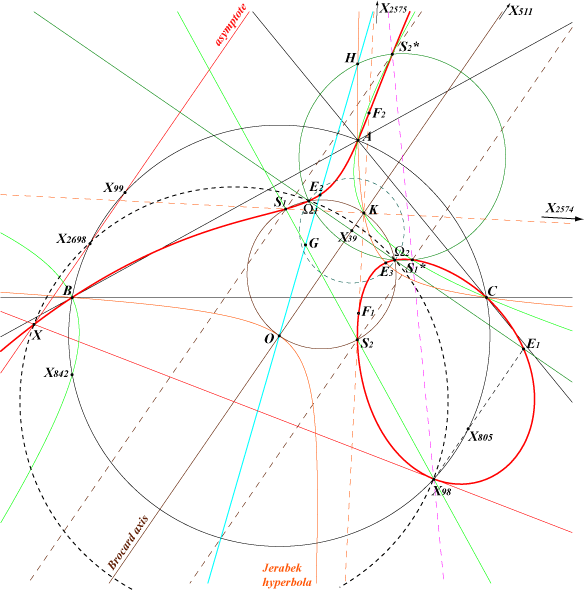Points on K019 K019 contains : • A, B, C. • the intersections of the sidelines of ABC and the trilinear polar of the root X(647). • X(511), the infinite point of the Brocard axis OK. The real asymptote is the parallel at the Steiner point X(99) to the Brocard axis. • two other points on this line OK which are imaginary and also lie on pK(X6, X511). • the circular points at infinity. • the Brocard points Ω1 and Ω2. • the orthogonal projection E1 = X(16068) of X(98) on the line through Ω1 and Ω2. • X(98), the singular focus. • X = X(16069) = isogonal conjugate of E1, on the tangent at X(98), on the real asymptote, and on the circle through X(98), Ω1 and Ω2. X is the common tangential of X(98), X(511) and the Brocard points. • the four foci of the "K-ellipse", the inconic with center K. • the four foci of the inconic with center O. • three points on the Euler line which also lie on Q002, the Euler-Morley quartic, one of them E2 = X(10221) is always real. • three points on the Jerabek hyperbola (apart A, B, C) which also lie on Q003, the Euler-Morley quintic, one of them E3 is always real. E3 is the isogonal conjugate of E2 = X(10221). • the six intersections of the altitudes and the cevian lines of O (Wilson Stothers). • two points S1, S2 on the line X(2)X(98) which are now X(13414), X(13415) in ETC (2017-05-22). See also Table 62. These points S1, S2 are always real and lie on the Brocard circle, the parallels at K and O to the asymptotes of the Jerabek hyperbola or to the axes of the K-ellipse, the parallels at X(1113), X(1114) to the Brocard axis. the McCay hessian cubic K048. • their isogonal conjugates S1*, S2* now X(16070), X(16071) which lie on the line through X(98) and X(648), the circum-hyperbola through X(6), X(232), X(250), X(262), X(264), X(325), X(511), X(523), X(842), X(1485), X(2065). the circle through H, Ω1 and Ω2. the parallels at S2, S1 to the Brocard axis. • T1 = X(98)Ω1 /\ X(511)Ω2 and its isogonal conjugate T2 = X(98)Ω2 /\ X(511)Ω1.Hessian and prehessians of K019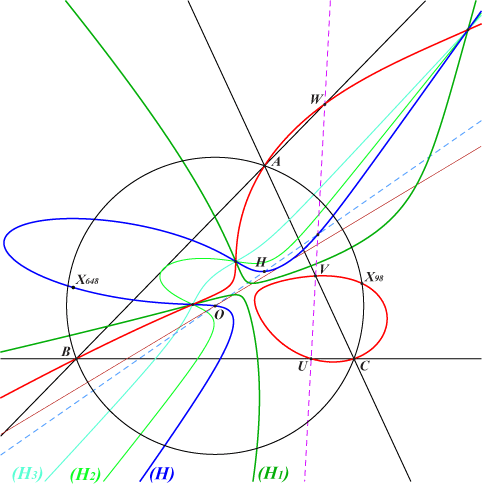The hessian (H) of K019 contains O, H, X(648), the intersection of the Euler line with the trilinear polar of the root X(647). Note that the polar conic of X(648) in K019 is the union of these two latter lines. K019 has always three real prehessians (H1), (H2), (H3) and one of them – say (H1) – is a stelloid with radial center X(98), the singular focus of K019. The cubics – apart (H1) – share the same orthic line, namely the Brocard axis. Each prehessian is associated with a natural conjugation Fi on K019 which swaps a point and the center of its polar conic in (Hi).Conjugations on K019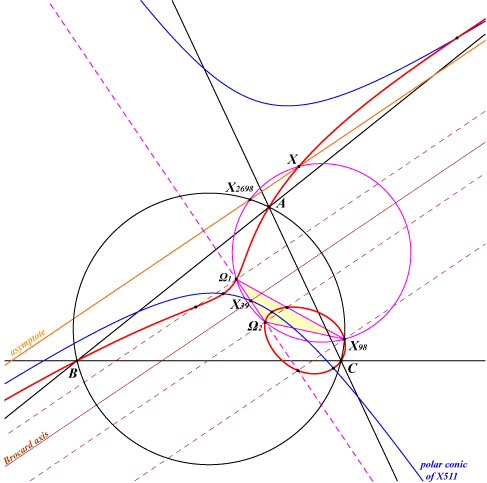K019 is the isogonal pK with pivot X(511) with respect to the triangle Ω1 Ω2 X(98). Hence, K019 contains the in/excenters of this latter triangle which are four centers of anallagmaty on the cubic. These points lie on the polar conic of X(511), a rectangular hyperbola with center X(2968), passing through X(39) and X(512). It follows that K019 is now invariant under ten conjugations namely : • isogonal conjugation in ABC, • isogonal conjugation in Ω1 Ω2 X(98), • transformation JS (see Table 62) which maps a point M to the intersections of the polar lines of M in the Jerabek and Stammler rectangular hyperbolas, • three involutions F1, F2, F3 above, • four inversions with center one of the in/excenters of triangle Ω1 Ω2 X(98) that swap another in/excenter and the corresponding vertex of triangle Ω1 Ω2 X(98).Two axial cubics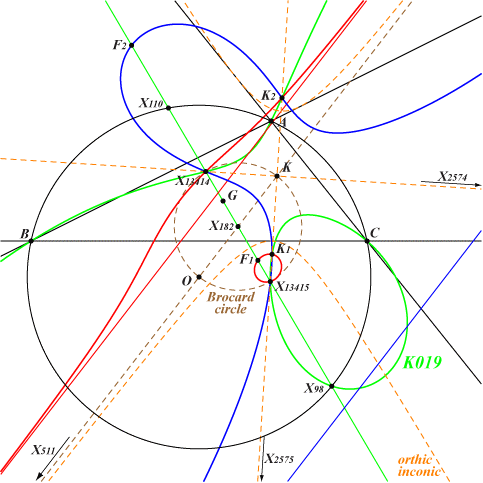K1, K2 are the real foci of the orthic inconic. F1, F2 are the singular foci of the two axial cubics. The respective tangents at these points are parallel to the Brocard axis.